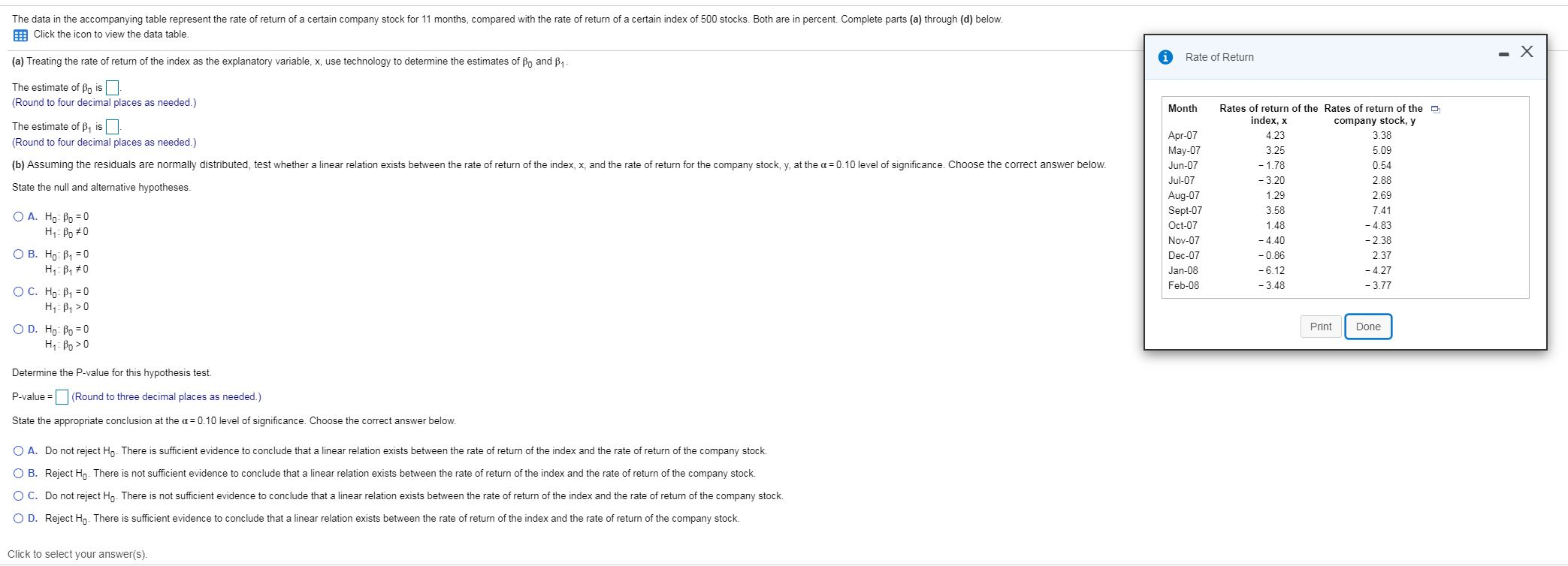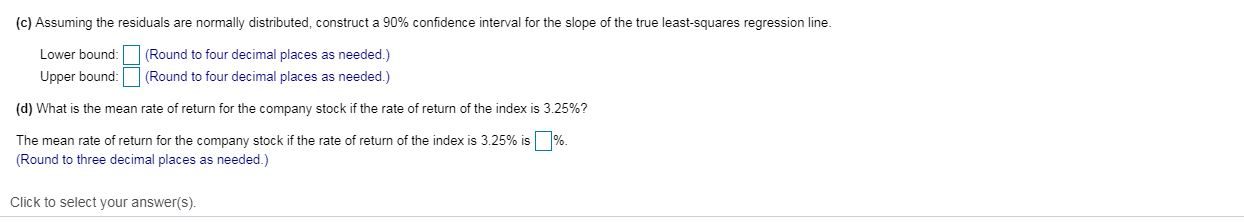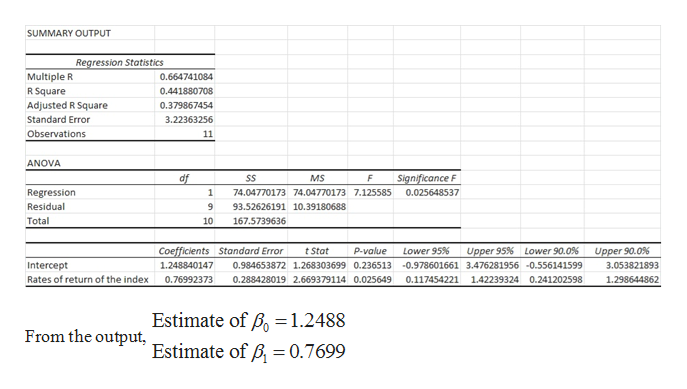# (c) Assuming the residuals are normally distributed, construct a 90% confidence interval for the slope of the true least-squares regression line.(Round to four decimal places as needed.)Lower bound:Upper bound(Round to four decimal places as needed.)(d) What is the mean rate of return for the company stock if the rate of return of the index is 3.25%?%.The mean rate of return for the company stock if the rate of return of the index is 3.25% is(Round to three decimal places as needed.)Click to select your answer(s).

Question
86 viewsfullscreenhelp_outlineImage Transcriptionclose(c) Assuming the residuals are normally distributed, construct a 90% confidence interval for the slope of the true least-squares regression line. (Round to four decimal places as needed.) Lower bound: Upper bound (Round to four decimal places as needed.) (d) What is the mean rate of return for the company stock if the rate of return of the index is 3.25%? %. The mean rate of return for the company stock if the rate of return of the index is 3.25% is (Round to three decimal places as needed.) Click to select your answer(s). fullscreen
check_circle

Step 1

Note:

Thank you for the question. As your question has more than 3 parts, according to our policy, we are answering the first 3 parts for you. If you need help with any other particular part, please re-post the question and mention the part you need help with.

Step 2

Part (a):

In order to obtain the least square regression line, first perform regression analysis on the data.

The regression analysis is conducted here by using EXCEL. The software procedure is given below:

• Enter the data.
• Select Data > Data Analysis > Regression> OK.
• Enter Input Y Range as \$B\$1:\$B\$12.
• Enter Input X Range as \$A\$1:\$A\$12.
• Click OK.

The output using EXCEL is as follows:help_outlineImage TranscriptioncloseSUMMARY OUTPUT Regression Statistics Multiple R R Square Adjusted R Square 0.664741084 0.441880708 0.379867454 Standard Error 3.22363256 Observations 11 ANOVA Significance F df SS MS Regression 1 74.04770173 74.04770173 7.125585 0.025648537 Residual 9 93.52626191 10.39180688 Total 10 167.5739636 Coefficients Standard Error Lower 95% Upper 95 % t Stat P-value Lower 90.0 % Upper 90.0 % 1.248840147 Intercept 0.984653872 1.268303699 0.236513 -0.978601661 3.476281956 -0.556141599 3.053821893 Rates of return of the index 0.288428019 2.669379114 0.025649 0.117454221 142239324 0.241202598 1.298644862 0.76992373 Estimate of Bo= 1.2488 Estimate of B 0.7699 From the output, fullscreen
Step 3

Part (b):

The aim of the test is to find whether there a linear relationship exist between rate of return of index and rate of return of the company stock.

The null hypothesis of the form:

H0: β1 = 0, that is there is no linear relationship exist between rate of return of index and rate of return of the company stock.

The alternative hypothesis is of the form:

H1: β1 ≠ 0, that is there is a linear relationship exists between rate of return of index and rate of return of the company stock.

Correct option is Option A.

Decision based on p-value:

At level of significance α, if the p-value ≤ α, then reject the null hypothesis, H0. Otherwise fail to reject H0.

From Part (a) outputs, t = 2.6694 and p-value =0.026.

Conclusion:

Here, p-value is...

### Want to see the full answer?

See Solution

#### Want to see this answer and more?

Solutions are written by subject experts who are available 24/7. Questions are typically answered within 1 hour.*

See Solution
*Response times may vary by subject and question.
Tagged in

### Probability